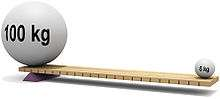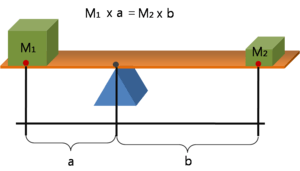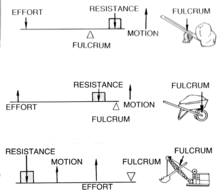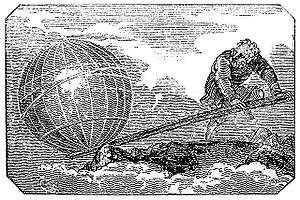# Lever

Lever, one of the six simple machinesLevers can be used to exert a large force over a small distance at one end by exerting only a small force over a greater distance at the other.
Classification Simple machine
Industry Construction
Weight Mass times gravitational acceleration
Fuel source potential and kinetic energy {mechanical energy }
Components fulcrum or pivot, load and effort

A lever (/ˈlvər/ or US /ˈlɛvər/) is a machine consisting of a beam or rigid rod pivoted at a fixed hinge, or fulcrum. A lever is a rigid body capable of rotating on a point on itself. On the basis of the location of fulcrum, load and effort, the lever is divided into three types. It is one of the six simple machines identified by Renaissance scientists. A lever amplifies an input force to provide a greater output force, which is said to provide leverage. The ratio of the output force to the input force is the mechanical advantage of the lever.

## Etymology

The word "lever" entered English about 1300 from Old French, in which the word was levier. This sprang from the stem of the verb lever, meaning "to raise". The verb, in turn, goes back to the Latin levare, itself from the adjective levis, meaning "light" (as in "not heavy"). The word's primary origin is the Proto-Indo-European (PIE) stem legwh-, meaning "light", "easy" or "nimble", among other things. The PIE stem also gave rise to the English word "light".

## Early use

The earliest remaining writings regarding levers date from the 3rd century BC and were provided by Archimedes. 'Give me a place to stand, and I shall move the Earth with it' is a remark of Archimedes who formally stated the correct mathematical principle of levers (quoted by Pappus of Alexandria). The distance required to do this might be exemplified in astronomical terms as the approximate distance to the Circinus galaxy (roughly 3.6 times the distance to the Andromeda Galaxy) - about 9 million light years.

It is assumed that in ancient Egypt, constructors used the lever to move and uplift obelisks weighing more than 100 tons.

## Force and leversA lever in balance

A lever is a beam connected to ground by a hinge, or pivot, called a fulcrum. The ideal lever does not dissipate or store energy, which means there is no friction in the hinge or bending in the beam. In this case, the power into the lever equals the power out, and the ratio of output to input force is given by the ratio of the distances from the fulcrum to the points of application of these forces. This is known as the law of the lever.

The mechanical advantage of a lever can be determined by considering the balance of moments or torque, T, about the fulcrum.where F1 is the input force to the lever and F2 is the output force. The distances a and b are the perpendicular distances between the forces and the fulcrum.

Since the moments of torque must be balanced,. So,.

The mechanical advantage of the lever is the ratio of output force to input force,This relationship shows that the mechanical advantage can be computed from ratio of the distances from the fulcrum to where the input and output forces are applied to the lever, assuming no losses due to friction, flexibility or wear.

## Classes of leversThree classes of levers

Levers are classified by the relative positions of the fulcrum, effort and resistance (or load). It is common to call the input force the effort and the output force the load or the resistance. This allows the identification of three classes of levers by the relative locations of the fulcrum, the resistance and the effort:

• Class 1: Fulcrum in the middle: the effort is applied on one side of the fulcrum and the resistance (or load) on the other side, for example, a seesaw, a crowbar or a pair of scissors. Mechanical advantage may be greater than, less than, or equal to 1.
• Class 2: Resistance (or load) in the middle: the effort is applied on one side of the resistance and the fulcrum is located on the other side, for example, a wheelbarrow, a nutcracker, a bottle opener or the brake pedal of a car. Mechanical advantage is always greater than 1.
• Class 3: Effort in the middle: the resistance (or load) is on one side of the effort and the fulcrum is located on the other side, for example, a pair of tweezers or the human mandible. Mechanical advantage is always less than 1.

These cases are described by the mnemonic fre 123 where the fulcrum is in the middle for the 1st class lever, the resistance is in the middle for the 2nd class lever, and the effort is in the middle for the 3rd class lever.

## Law of the lever

The lever is a movable bar that pivots on a fulcrum attached to a fixed point. The lever operates by applying forces at different distances from the fulcrum, or a pivot.

Assuming the lever does not dissipate or store energy, the power into the lever must equal the power out of the lever. As the lever rotates around the fulcrum, points farther from this pivot move faster than points closer to the pivot. Therefore, a force applied to a point farther from the pivot must be less than the force located at a point closer in, because power is the product of force and velocity.

If a and b are distances from the fulcrum to points A and B and the force FA applied to A is the input and the force FB applied at B is the output, the ratio of the velocities of points A and B is given by a/b, so we have the ratio of the output force to the input force, or mechanical advantage, is given byThis is the law of the lever, which was proven by Archimedes using geometric reasoning. It shows that if the distance a from the fulcrum to where the input force is applied (point A) is greater than the distance b from fulcrum to where the output force is applied (point B), then the lever amplifies the input force. On the other hand, if the distance a from the fulcrum to the input force is less than the distance b from the fulcrum to the output force, then the lever reduces the input force.

The use of velocity in the static analysis of a lever is an application of the principle of virtual work.

## Virtual work and the law of the lever

A lever is modeled as a rigid bar connected to a ground frame by a hinged joint called a fulcrum. The lever is operated by applying an input force FA at a point A located by the coordinate vector rA on the bar. The lever then exerts an output force FB at the point B located by rB. The rotation of the lever about the fulcrum P is defined by the rotation angle θ in radians.This is an engraving from Mechanics Magazine published in London in 1824.

Let the coordinate vector of the point P that defines the fulcrum be rP, and introduce the lengthswhich are the distances from the fulcrum to the input point A and to the output point B, respectively.

Now introduce the unit vectors eA and eB from the fulcrum to the point A and B, soThe velocity of the points A and B are obtained aswhere eA and eB are unit vectors perpendicular to eA and eB, respectively.

The angle θ is the generalized coordinate that defines the configuration of the lever, and the generalized force associated with this coordinate is given bywhere FA and FB are components of the forces that are perpendicular to the radial segments PA and PB. The principle of virtual work states that at equilibrium the generalized force is zero, that isThus, the ratio of the output force FB to the input force FA is obtained aswhich is the mechanical advantage of the lever.

This equation shows that if the distance a from the fulcrum to the point A where the input force is applied is greater than the distance b from fulcrum to the point B where the output force is applied, then the lever amplifies the input force. If the opposite is true that the distance from the fulcrum to the input point A is less than from the fulcrum to the output point B, then the lever reduces the magnitude of the input force.Wikimedia Commons has media related to Levers.Look up lever in Wiktionary, the free dictionary.